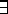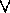Abstracts of Proof Theory Papers

On Goal-Directed Provability in Classical Logic

James Harland

to appear in a Special Issue of Computer Languages

One of the key features of logic programming is the notion of goal-directed provability. In intuitionistic logic, the notion of uniform proof has been used as a proof-theoretic characterization of this property. Whilst the connections between intuitionistic logic and computation are well known, there is no reason per se why a similar notion cannot be given in classical logic. In this paper we show that there are two notions of goal-directed proof in classical logic, both of which are suitably weaker than that for intuitionistic logic. We show the completeness of this class of proofs for certain fragments, which thus form logic programming languages. As there are more possible variations on the notion of goal-directed provability in classical logic, there is a greater diversity of classical logic programming languages than intuitionistic ones. In particular, we show how logic programs may contain disjunctions in this setting. This provides a proof-theoretic basis for disjunctive logic programs, as well as characterising the ``disjunctive'' nature of answer substitutions for such programs in terms of the provability properties of the classical connectivesand.

A Proof-Theoretic Analysis of Goal-Directed Provability

James Harland

Journal of Logic and Computation 4:1:69-88, January, 1994.

Uniform proofs have been presented as a basis for logic programming, and it is known that by restricting the class of formulae it is possible to guarantee that uniform proofs are complete with respect to provability in intuitionistic logic. In this paper we explore the relationship between uniform proofs and classes of formulae more deeply. Firstly we show that uniform proofs arise naturally as a normal form for proofs in first-order intuitionistic sequent calculus. Next we show that the class of formulae known as hereditary Harrop formulae are intimately related to uniform proofs, and that we may extract such formulae from uniform proofs in two different ways. We also give results which may be interpreted as showing that hereditary Harrop formulae are the largest class of formulae for which uniform proofs are guaranteed to be complete. Finally we briefly discuss some possibilities for a slightly more general approach using intermediate and infinitary logics. Paper

Success and Failure for Hereditary Harrop Formulae

James Harland

Journal of Logic Programming 17:1:1-29, October, 1993.

We introduce the foundational issues involved in incorporating the Negation as Failure (NAF) rule into the framework of first-order hereditary Harrop formulae of Miller et al. This is a larger class of formulae than Horn clauses, and so the technicalities are more intricate than in the Horn clause case. As programs may grow during execution in this framework, the role of NAF and the Closed World Assumption (CWA) need some modification, and for this reason we introduce the notion of a completely defined predicate, which may be thought of as a localisation of the CWA. We also show how this notion may be used to define a notion of NAF for a more general class of goals than literals alone. We also show how an extensional notion of universal quantification may be incorporated. This makes our framework somewhat different from that of Miller et al., but not essentially so. We also show how to construct a Kripke-like model for the extended class of programs. This is essentially a denotational semantics for logic programs, in that it provides a mapping from the program to a pair of sets of atoms which denote the success and (finite) failure sets. This is inspired by the work of Miller on the semantics of first-order hereditary Harrop formulae. Note that no restriction on the class of programs is needed in this approach, and that our construction needs no more than omega iterations. This necessitates a slight departure from the standard methods, but the important properties of the construction still hold. Paper

Structural Properties of Logic Programs

James Harland

Proceedings of the Fourteenth Australian Computer Science Conference, Sydney, February, 1991. Published as Australian Computer Science Communications:13:1, 1991.

Miller has shown that disjunctions are not necessary in a large fragment of hereditary Harrop formulae, a class of formulae which properly includes Horn clauses. In this paper we extend this result to include existential quantifications, so that for each program P, there is a program P' which is operationally equivalent, but contains no disjunctions or existential quantifications. We may think of this process as deriving a normal form for the program. This process is carried out by pushing the connectives outwards from the body of a clause, and this process leads to a normal form for goals as well. The properties of the search process used to find uniform proofs of goals (which generalises SLD-resolution) together with the normal form allow successful goals to be converted into program clauses, and so we may add successful goals to the program. The stored form of the goal requires a larger class of formulae, i.e. full first-order hereditary Harrop formulae, and so this leads to a natural separation into an object level and a meta-level. This separation seems intuitively natural and to correspond to aspects of programming methodology. Finally we discuss some possibilities for amalgamation of the object and meta-level languages. Paper

A Clausal Form for the Completion of Logic Programs

James Harland

Proceedings of the International Conference on Logic Programming 711-725, Paris, June, 1991.

The Clark completion of a program is a way of making explicit the inferences which may be made from the program using the Negation as Failure (NAF) rule. This may be thought of as adding negative information to the program in such a way that an atom fails iff its negation is derivable from the completion of the program. We show how the completion process may be extended to hereditary Harrop formulae, a class of formulae that properly includes Horn clauses, and, more importantly, that the completion of a hereditary Harrop formula program may be expressed as a hereditary Harrop formula program. In fact, we show there are two ways to perform such a completion: one way which preserves inference, and another in which inconsistencies are detected. Moreover, we show that the two notions coincide for locally consistent programs, a class which properly includes locally stratified programs. In addition, we show how the completion may be used to generate complementary definitions under some circumstances, so that predicates such as odd and even may be synthesised from each other. Paper

A Proof-theoretic Analysis of Goal-Directed Provability

James Harland

Proceedings of the Fifteenth Australasian Computer Science Conference 337-349, Hobart, January, 1992.

One of the distinguishing features of logic programming seems to be the notion of goal-directed provability, i.e. that the structure of the goal is used to determine the next step in the proof search process. It is known that by restricting the class of formulae it is possible to guarantee that a certain class of proofs, known as uniform proofs, are complete with respect to provability in intuitionistic logic. In this paper we explore the relationship between uniform proofs and classes of formulae more deeply. Firstly we show that uniform proofs arise naturally as a normal form for proofs in first-order intuitionistic sequent calculus. Next we show that the class of formulae known as hereditary Harrop formulae are intimately related to uniform proofs, and that we may extract such formulae from uniform proofs in two different ways. We also give results which may be interpreted as showing that hereditary Harrop formulaeare the largest class of formulae for which uniform proofs are guaranteed to be complete, along the lines of an interpolation theorem. Paper

On Normal Forms and Equivalence for Logic Programs

James Harland

Proceedings of the Joint International Conference and Symposium on Logic Programming 146-160, Washington DC, November, 1992.

It is known that larger classes of formulae than Horn clauses may be used as logic programming languages. One such class of formulae is hereditary Harrop formulae, for which an operational notion of provability has been studied, and it is known that operational provability corresponds to provability in intuitionistic logic. In this paper we discuss the notion of a normal form for this class of formulae, and show how this may be given by removing disjunctions and existential quantifications from programs. Whilst the normal form of the program preserves operational provability, there are operationally equivalent programs which are not intuitionistically equivalent. As it is known that classical logic is too strong to precisely capture operational provability for larger classes of programs than Horn clauses, the appropriate logic in which to study questions of equivalence is an intermediate logic. We explore the nature of the required logic, and show that this may be obtained by the addition of the Independence of Premise axioms to intuitionistic logic. We show how equivalence in this logic captures the notion of operational provability, in that logically equivalent programs are operationally equivalent. This result suggests that the natural logic in which to study logic programming is a slightly stronger constructive logic than intuitionistic logic. Paper

On Goal-Directed Provability in Classical Logic

James Harland

Proceedings of the Workshop on Proof-Theoretical Extensions of Logic Programming 10-18, Santa Marghertia Ligure, June, 1994.

Towards a Sequent Calculus for Negation as Failure

James Harland

Proceedings of the Workshop on Proof-Theoretical Extensions of Logic Programming 19-27, Santa Marghertia Ligure, June, 1994.